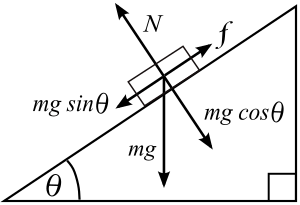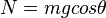# Inclined plane facts for kids

Kids Encyclopedia Facts

An inclined plane is a simple machine. It allows one to use less force to move an object.

Examples of inclined planes are ramps, sloping roads and hills, plows, chisels, hatchets, carpenter's planes, and wedges. The typical example of an inclined plane is a sloped surface; for example a roadway to bridge at a different height.

Another simple machine based on the inclined plane is the blade, in which two inclined planes placed back to back allow the two parts of the cut object to move apart using less force than would be needed to pull them apart in opposite directions.

## Calculation of forces acting on an object on an inclined planesKey:
N = Normal force that is perpendicular to the plane
m = Mass of object
g = Acceleration due to gravity
θ (theta) = Angle of elevation of the plane, measured from the horizontal
f = frictional force of the inclined plane

To calculate the forces on an object placed on an inclined plane, consider the three forces acting on it.

1. The normal force (N) exerted on the body by the plane due to the attraction of gravity i.e. mg cos θ
2. the force due to gravity (mg, acting vertically downwards) and
3. the frictional force (f) acting parallel to the plane.

We can break the force from gravity into two vectors, one perpendicular to the plane and one parallel to the plane. Since there is no movement perpendicular to the plane, the component of the gravitational force in this direction (mg cos θ) must be equal and opposite to normal force exerted by the plane, N. Therefore,$N=mgcos\theta$.

If the component of the force from gravity parallel to the surface (mg sin θ) is greater than the static frictional force fs – then the body will slide down the inclined plane with acceleration (g sin θfk/m), where fk is the force of friction – otherwise it will remain stationary.

When the angle of the slope (θ) is zero, sin θ is also zero, so the body will not move.

## Images for kidsInclined plane Facts for Kids. Kiddle Encyclopedia.